PhysicsShowing results 41 to 50 of 88, on page 5 of 9 Electricity and Magnetism Mechanics Modern Physics Optics Oscillations and Waves Thermodynamics
 DESCRIPTION EQUATION Kinetic energy of simple harmonic motion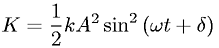Magnetic dipole moment of a current loop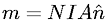Magnetic field inside a solenoid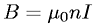Magnetic flux definedMagnetic force on a moving chargeMaxwell's equation - Faraday's law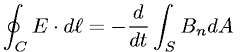Maxwell's equation - Gauss's law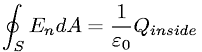Net power radiated by an object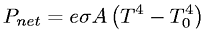Newton's Second Law (Force)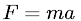Number density of charge carriers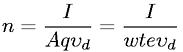Go to page: 1  2  3  4  5  6  7  8  9